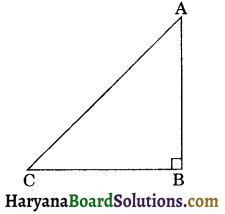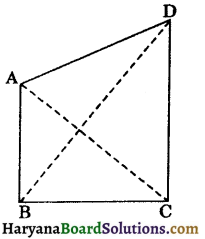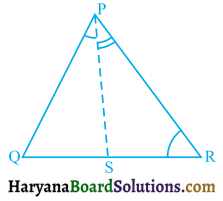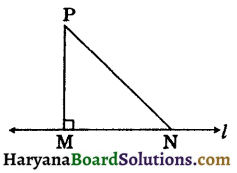# HBSE 9th Class Maths Solutions Chapter 7 Triangles Ex 7.4

Haryana State Board HBSE 9th Class Maths Solutions Chapter 7 Triangles Ex 7.4 Textbook Exercise Questions and Answers.

## Haryana Board 9th Class Maths Solutions Chapter 7 Triangles Exercise 7.4

Question 1.
Show that in a right-angled triangle, the hypotenuse is the longest side.
Solution :
Given: A right-angled ΔABC in which ∠B = 90°
To prove: Hypotenuse is the longest side i.e.
(i) AC > BC
(ii) AC > AB.Proof: (i) In ΔABC, we have
∠B = 90°
But we know that sum of interior angles of a triangle is 180°
∴ ∠A + ∠B + ∠C = 180°
⇒ ∠A + 90° + ∠C = 180°
⇒ ∠A + ∠C = 180° – 90° = 90°
⇒ ∠A and ∠C are acute angles
⇒ ∠B > ∠A
⇒ AC > BC,
(Side opposite to greater angle is larger)

(ii) Similarly. ∠B > ∠C
⇒ AC > AB
Hence, hypotenuse is the longest side.
ProvedQuestion 2.
In the figure 7.73, sides AB and AC of AABC are extended to points P and Q respectively. Also, ∠PBC < ∠QCB. Show that AC > ABSolution:
In ΔABC, we have
∠PBC = ∠BAC + ∠ACB …(i)
and ∠QCB = ∠BAC + ∠ABC …(ii)
Now, ∠PBC < ∠QCB (Given)
⇒ ∠BAC + ∠ACB < ∠BAC + ∠ABC [Using (i) and (ii)]
⇒ ∠ACB < ∠ABC ⇒ ∠ABC > ∠ACB
⇒ AC > AB,
(side opposite to greater angle is larger)
Hence proved

Question 3.
In figure ∠B < ∠A and ∠C < ∠D. Show that AD < BC.Solution:
In ΔAOB
∠B < ∠A, (Given) ⇒ ∠A > ∠B
⇒ BO > AO …..(i)
(Side opposite to greater angle is larger)
In ΔCOD, ∠C > ∠D, (Given)
⇒ ∠D > ∠C
⇒ OC > OD ….(ii)
(Side opposite to greater angle is larger)
Adding (i) and (ii), we get
BO + OC > AO + OD
Hence provedQuestion 4.
AB and CD are respectively the smallest and longest sides of a quadrilateral ABCD (see figure 7.75). Show that ∠A > ∠C and ∠B > ∠D (NCERT Exemplar Problems]Solution :
Join AC and BD.
In ΔABC, BC > AB
(∵ AB is the smallest side)
⇒ ∠BAC > ∠ACB ………(i)
(Angle opposite to greater side is larger)
[∵ CD is the largest side]
[Angle opposite to greater side is larger]
Adding (i) and (ii), we get∠BAC + ∠CAD > ∠ACB + ∠ACD
⇒ ∠A > ∠C
(∵ AB is the smallest side)
⇒ ABD > ZADB …(iii) [Angle opposite to longer side is larger]
In ΔBCD, CD > BC,
(∵ CD is the greatest side)
⇒ ∠CBD > ∠CDB …(iv) (Angle opposite to longer side is larger)
Adding (iii) and (iv), we get
∠ABD + ∠CBD > ∠ADB + ∠CDB
⇒ ∠B > ∠D
Hence, ∠A > ∠C and ∠B > ∠D.
Hence Proved

Question 5.
In figure 7.77, PR > PQ and PS bisects ∠QPR. Prove that ∠PSR > ∠PSQ.Solution:
In ΔPQR, PR > PQ. (Given)
⇒ ∠PQR > ∠PRQ ……(i)
[∵ Angle opposite to longer side is larger]
⇒ ∠PQR + ∠QPS > ∠PRQ + ∠QPS,
⇒ ∠PQR + ∠QPS > ∠PRQ + ∠RPS,
∵ PS is the bisector of ∠QPR
∴ ∠QPS = ∠RPS
⇒ ∠PQS + ∠QPS > ∠PRS + ∠RPS,
[∵ ∠PQR = ∠PQS
and ∠PRQ = ∠PRS] …… (ii)
In ΔPQS, we have
∠PSR = ∠PQS + ∠QPS, …(iii)
(By theorem 6.8)
In ΔPRS, we have
∠PSQ = ∠PRS + ∠RPS, …(iv)
(By theorem 6.8)
From (ii), (iii) and (iv), we get
∠PSR > ∠PSQ. Hence provedQuestion 6.
Show that of all line segments drawn from a given point not on it, the perpendicular line segment is the shortest.
Solution:
Given: l is a line and P is a point not lying on it. PM ⊥ l and N is any point on l other than M.
To prove: PM < PN.Proof: In ΔPNM,
∠PMN = 90°, (∵ PM ⊥ l)
∴ ∠PNM + ∠MPN = 90°
⇒ ∠PNM < 90°
⇒ ∠PNM < ∠PMN ⇒ ∠PMN >∠PNM
⇒ PN > PM (Side opposite to greater angle is longer)
⇒ PM